# Databases - Online Test

Q1. Which of the following statements are TRUE about an SQL query? P : An SQL query can contain a HAVING clause even if it does not have a GROUP BY clause Q : An SQL query can contain a HAVING clause only if it has GROUP BY clause R : All attributes used in the GROUP BY clause must appear in the SELECT clause S : Not all attributes used in the GROUP BY clause need to appear in the SELECT clause
Explaination / Solution:

If we use a HAVING clause without a GROUP BY clause, the HAVING condition applies to all rows that satisfy the search condition. In other words, all rows that satisfy the search condition make up a single group. So, option P is true and Q is false.
S is also true as an example consider the following table and query.Q2. Given the basic ER and relational models, which of the following is INCORRECT?
Explaination / Solution:

The term ‘entity’ belongs to ER model and the term ‘relational table’ belongs to relational model. Options A and B both are true since ER model supports both multivalued and composite attributes. As multivalued attributes are not allowed in relational databases, in a row of a relational (table), an attribute cannot have more than one value.

Q3. Which of the following is TRUE?
Explaination / Solution:

Option A is false since BCNF is stricter than 3NF (it needs LHS of all FDs should be candidate key for 3NF condition) Option B is false since the definition given here is of 2NF Option C is true, since for a relation to be in BCNF it needs to be in 3NF, every relation in BCNF satisfies all the properties of 3NF. Option D is false, since if a relation is in BCNF it will always be in 3NF.

Q4. Suppose R1are two relation schemas. Let r1 and r2 be the corresponding relation instances. B is a foreign key that refers to C in R2. If data in r1 and r2 satisfy referential integrity constrains, which of the following is ALWAYS TRUE?
Explaination / Solution:
No Explaination.

Q5. Consider the following relations A, B and C: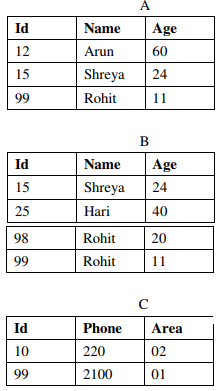How many tuples does the result of the following SQL query contain?
SELECT A.Id
FROM A
WHERE A.Age > ALL(SELECT B.Age FROM B WHERE B.Name = ‘Arun’)
Explaination / Solution:

As the result of subquery is an empty table, ‘>ALL’ comparison is true . Therefore, all the three row id’s of A will be selected from table A.

Q6. Consider the following relations A, B and C:How many tuples does the result of the following relational algebra expression contain? Assume that the schema of A ∪ B is the same as that of A.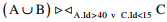Explaination / Solution:Q7. Which of the following assertions are CORRECT?
P: Adding 7 to each entry in a list adds 7 to the mean of the list
Q: Adding 7 to each entry in a list adds 7 to the standard deviation of the list
R: Doubling each entry in a list doubles the mean of the list
S: Doubling each entry in a list leaves the standard deviation of the list unchanged
Explaination / Solution:

P and R always hold true Else consider a sample set {1, 2, 3, 4} and check accordingly

Q8. An index is clustered, if
Explaination / Solution:

Clustered index is built on ordering non key field and hence if the index is clustered then the data records of the file are organized in the same order as the data entries of the index.

Q9. Using public key cryptography, X adds a digital signature σ to message M, encrypts , and sends it to Y, where it is decrypted. Which one of the following sequences of keys is used for the operations?
Explaination / Solution: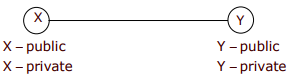Encryption: Source has to encrypt with its p r ivate key for formin g Digital signature for Authentication. source has to encrypt the <M, σ > with Y ' s public key to send it confidentially
Decryption: Destination Y has to decrypt first with its private key, then decrypt using source public key

Q10.
Consider the following relational schema.
Students(rollno: integer, sname: string)
Courses(courseno: integer, cname: string)
Registration(rollno: integer, courseno; integer, percent: real)
Which of the following queries are equivalent to this query in English?
“Find the distinct names of all students who score more than 90% in the course numbered 107”
(I)  SELECT DISTINCT S.sname FROM Students as S, Registration as R WHERE R.rollno=S.rollno AND R.Courseno=107 AND R.percent>90
(II)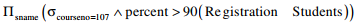(III) {T | ∃S∈ Students, ∃R∈ Registration (S.rollno = R.rollno ∧ R.courseno = 107 ∧ R.percent > 90 ∧ T.sname = S.name)}
(IV)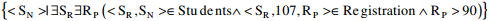Explaination / Solution:

Four queries given in SQL, RA, TRC and DRC in four statements respectively retrieve the required information.